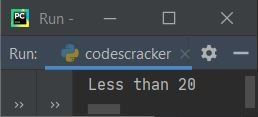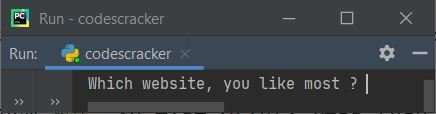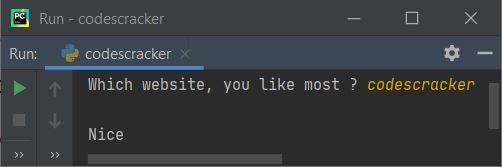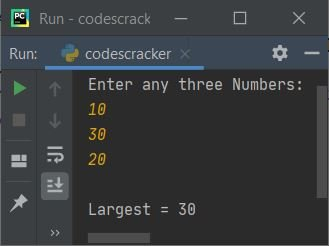# Python Ternary Operator with Examples

In this post, you'll learn all about the ternary operator along with an example. This post deals with the simple ternary operator as well as the nested ternary operator.

## What is the Ternary Operator in Python?

In Python, the ternary operator is essentially a shortened version of the if-else statement. That is, using the ternary operator, we can write at least four lines of code (using if-else) in only one line (using the ternary operator).

## Ternary Operator Syntax

The syntax to use the ternary operator in Python is a little different from other programming languages, but it is more clear and understandable, just like normal English. Here is its syntax:

`x if expression else y`

This is like the normal English we use, that is, evaluate x if the expression evaluates to be true, otherwise (else) evaluate y. Therefore, the above syntax can also be written like this:

`on_true if expression else on_false`

For example:

`print("10") if val==10 else print("Not 10")`

The above code prints 10 on the output if the value of the variable val is equal to 10, otherwise it prints "Not 10."

#### How can multiple lines of code be replaced with a single line?

Of course, this can be done using the ternary operator. Here is a code that will replace multiple lines with a single line using the ternary operator:

```num = 10
if num > 20:
print("Greater than 20")
else:
print("Less than 20")```

The output produced by the above Python program is:The above program can be replaced using the ternary operator as shown in the program given below:

```num = 10
print("Greater than 20") if num > 20 else print("Less than 20")```

As you can see, four lines of code get replaced with a single line. Now you can understand how useful the ternary operator is.

## Python Ternary Operator Examples

I know, until now, you understood about the ternary operator. But let me show you some more varieties of ternary operators in Python. This helps you understand it more clearly.

```print("Which website, you like most ? ", end="")
web = input()
print("\nNice") if web=="codescracker" else print("\nOps!")```

Here is the initial sample output produced by the above Python program on the ternary operator:Now supply the input, say "codescracker," and press the ENTER key to see the output as shown in the snapshot given below:Here is another ternary operator example program that shows the most common use of the Python ternary operator:

```print("Enter any two Numbers: ")
num_one = int(input())
num_two = int(input())
largest = num_one if num_one > num_two else num_two
print("The largest number is:", largest)```

Here is its sample run with user inputs 10 and 20:## Nested ternary operator

A ternary operator inside another ternary operator is known as a "nested ternary operator. This is not limited to using only one ternary operator inside another. You can nest multiple ternary operators in one single line of code, of course.

#### Nested Ternary Operator Syntax

The syntax to use the nested ternary operator is:

`(on_true if expression else on_false) if expression else (on_true if expression else on_false)`

Don't worry (if you have no idea how it works), follow the example given below and its brief step-by-step description. You will understand how it works.

### Nested Ternary Operator Example

The famous example of using a nested ternary operator is to find the largest or smallest of three numbers. This time, I'm going to create a program using the nested ternary operator that ffinds and prints the largest of three given numbers:

```print("Enter any three Numbers: ")
a = int(input())
b = int(input())
c = int(input())

largest = (a if a > c else c) if a > b else (b if b > c else c)
print("\nLargest =", largest)```

Here is its sample run with user inputs 10, 30, and 20:Let's briefly explain the nested ternary operator from the above program. I'm sure that after reading all the lines given below that explain the nested ternary operator carefully, you'll get a complete understanding of the both ternary and nested ternary operators. Now, from the above program, the following code:

`(a if a > c else c) if a > b else (b if b > c else c)`

gets evaluated in a way that:

• First, `a > b` gets evaluated.
• Now, whether the left ternary operator statement gets evaluated or the right one gets evaluated, is totally dependent on the boolean result of the expression "a > b."
• That is, if it evaluates to true, then the left one gets evaluated.
• Otherwise, the right ternary operator statement gets evaluated.
• Since with the sample run provided above, the expression a > b or 10 > 30 evaluates to false.
• Therefore, the right ternary operator statement gets evaluated, and the left one gets skipped.
• The right ternary operator statement is `(b if b > c else c)`.
• In this statement again, if the expression `b > c` evaluates to true, therefore b will be the final result of whole statement.
• Otherwise, c will be the final result of the whole statement.
• But in this case, since the expression b > c or 30 > 20 evaluates to true
• Therefore, b will be the final result of the whole statement.
• And b's value, which is 30, gets initialized to the variable named largest.
• In this way, the nested ternary operator in Python works.

Note: Just remember one thing: true for left, false for right. That is, if the expression evaluates to true, then the left one gets evaluated, otherwise the right one gets evaluated.

Python Online Test

« Previous Tutorial Next Tutorial »